## ML Aggarwal Class 10 Solutions for ICSE Maths Chapter 4 Linear Inequations Chapter Test

These Solutions are part of ML Aggarwal Class 10 Solutions for ICSE Maths. Here we have given ML Aggarwal Class 10 Solutions for ICSE Maths Chapter 4 Linear Inequations Chapter Test

More Exercises

Question 1.
Solve the inequation : 5x – 2 ≤ 3(3 – x) where x ∈ { – 2, – 1, 0, 1, 2, 3, 4}. Also represent its solution on the number line.
Solution:
5x – 2 < 3(3 – x)
⇒ 5x – 2 ≤ 9 – 3x
⇒ 5x + 3x ≤ 9 + 2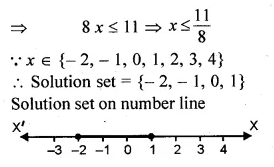Question 2.
Solve the inequations :
6x – 5 < 3x + 4, x ∈ I.
Solution:
6x – 5 < 3x + 4
6x – 3x < 4 + 5
⇒ 3x <9
⇒ x < 3
x ∈ I
Solution Set = { -1, -2, 2, 1, 0….. }

Question 3.
Find the solution set of the inequation
x + 5 < 2 x + 3 ; x ∈ R
Graph the solution set on the number line.
Solution:
x + 5 ≤ 2x + 3
x – 2x ≤ 3 – 5
⇒ -x ≤ -2
⇒ x ≥ 2Question 4.
If x ∈ R (real numbers) and – 1 < 3 – 2x ≤ 7, find solution set and represent it on a number line.
Solution:
-1 < 3 – 2x ≤ 7
-1 < 3 – 2x and 3 – 2x ≤ 7
⇒ 2x < 3 + 1 and – 2x ≤ 7 – 3
⇒ 2x < 4 and -2x ≤ 4
⇒ x < 2 and -x ≤ 2
and x ≥ -2 or -2 ≤ x
x ∈ R
Solution set -2 ≤ x < 2
Solution set on number line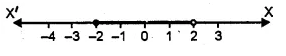Question 5.
Solve the inequation :
$$\frac { 5x+1 }{ 7 } -4\left( \frac { x }{ 7 } +\frac { 2 }{ 5 } \right) \le 1\frac { 3 }{ 5 } +\frac { 3x-1 }{ 7 } ,x\in R$$
Solution:
$$\frac { 5x+1 }{ 7 } -4\left( \frac { x }{ 7 } +\frac { 2 }{ 5 } \right) \le 1\frac { 3 }{ 5 } +\frac { 3x-1 }{ 7 }$$
$$\frac { 5x+1 }{ 7 } -4\left( \frac { x }{ 7 } +\frac { 2 }{ 5 } \right) \le \frac { 8 }{ 5 } +\frac { 3x-1 }{ 7 }$$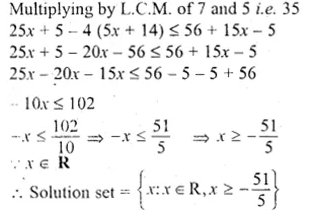Question 6.
Find the range of values of a, which satisfy 7 ≤ – 4x + 2 < 12, x ∈ R. Graph these values of a on the real number line.
Solution:
7 < – 4x + 2 < 12
7 < – 4x + 2 and – 4x + 2 < 12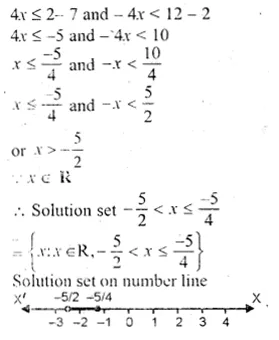Question 7.
If x∈R, solve $$2x-3\ge x+\frac { 1-x }{ 3 } >\frac { 2 }{ 5 } x$$
Solution:
$$2x-3\ge x+\frac { 1-x }{ 3 } >\frac { 2 }{ 5 } x$$
$$2x-3\ge x+\frac { 1-x }{ 3 }$$ and $$x+\frac { 1-x }{ 3 } >\frac { 2 }{ 5 } x$$Question 8.
Find positive integers which are such that if 6 is subtracted from five times the integer then the resulting number cannot be greater than four times the integer.
Solution:
Let the positive integer = x
According to the problem,
5a – 6 < 4x
⇒ 5a – 4x < 6
⇒ x < 6
Solution set = {x : x < 6}
= { 1, 2, 3, 4, 5, 6}

Question 9.
Find three smallest consecutive natural numbers such that the difference between one-third of the largest and one-fifth of the smallest is at least 3.
Solution:
Let first least natural number = x
then second number = x + 1
and third number = x + 2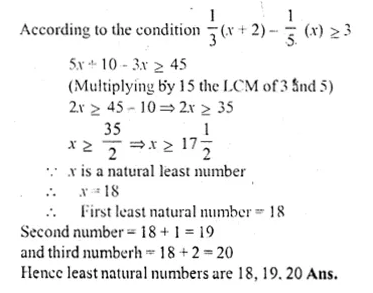Hope given ML Aggarwal Class 10 Solutions for ICSE Maths Chapter 4 Linear Inequations Chapter Test are helpful to complete your math homework.

If you have any doubts, please comment below. Learn Insta try to provide online math tutoring for you.